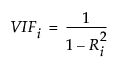Fitting Linear Models > Generalized Regression Models > Model Fit Reports > Parameter Estimates for Original Predictors
Publication date: 05/24/2021

##Parameter Estimates for Original Predictors

The Parameter Estimates for Original Predictors report gives estimates and other results for all parameters in the model. The initial table includes the coefficients for the predictors in the model. An additional table includes other model parameters such as scale, dispersion, or zero inflation parameters. See Distribution. Both tables include the same columns of results.

Tip: You can click terms in the Parameter Estimates for Original Predictors report to highlight the corresponding paths in the Solution Path Plot. The corresponding columns in the data table are also selected. This is useful when running further analyses. Press Shift and click the terms to select multiple rows.

The Parameter Estimates for Original Predictors report gives parameter estimates for the model expressed in terms of the original (uncentered and unscaled) predictors.

The report provides the following information:

Term

A list of the model terms. “Forced in” appears next to any terms that were forced into the model using the Advanced Controls option.

Estimate

The parameter estimate corresponding to the model term given in terms of the original measurements.

Std Error

The standard error of the estimate. This is obtained using M-estimation and a sandwich formula (Zou 2006 and Huber and Ronchetti 2009).

Wald ChiSquare

The ChiSquare value for a Wald test of whether the parameter is zero.

Prob > ChiSquare

The p-value for the Wald test.

Lower 95%

The lower bound for a 95% confidence interval for the parameter. You can change the α level in the Fit Model window by selecting Set Alpha Level from the Model Specification red triangle menu.

Upper 95%

The upper bound for a 95% confidence interval for the parameter. You can change the α level in the Fit Model window by selecting Set Alpha Level from the Model Specification red triangle menu.

Singularity Details

(Available only if there are linear dependencies among the model terms.) The linear function that the model term satisfies.

VIF

(Available only when the distribution is Normal. Appears only if you right-click in the parameter estimates table and select Columns > VIF.) The variance inflation factor (VIF) for each term in the model. High VIF values indicate a collinearity issue among the terms in the model.

The VIF for the ith term, xi, is calculated as follows:where Ri 2 is the RSquare for the regression of xi as a function of the other explanatory variables.